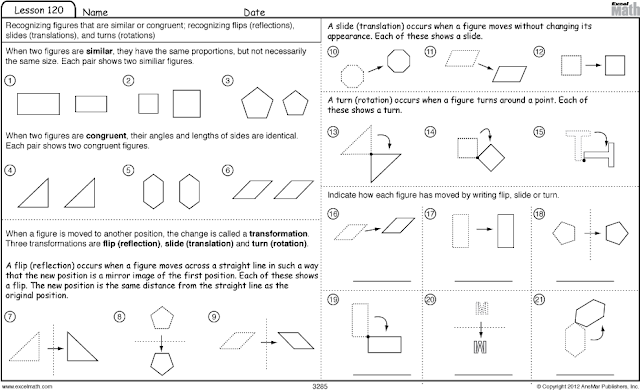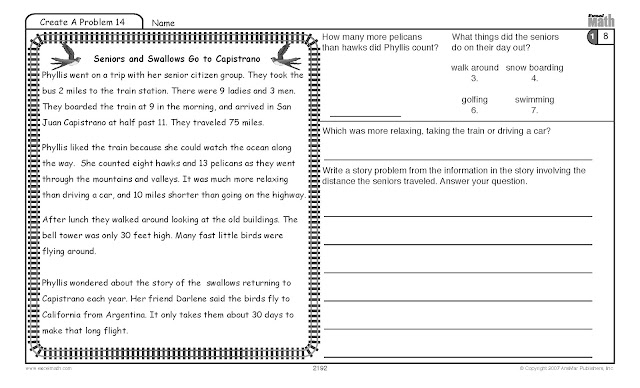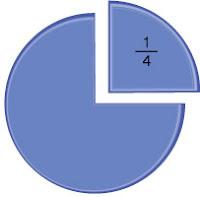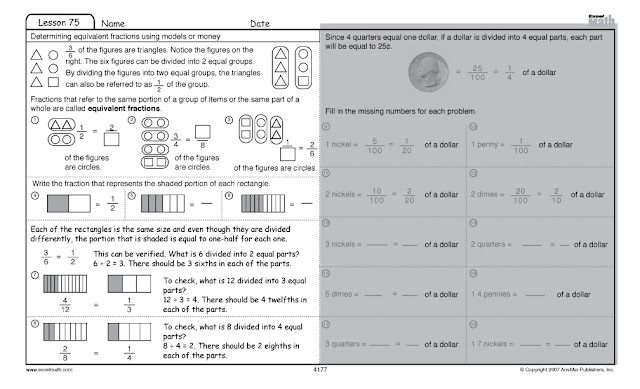## Monday, April 30, 2012

### Slide, Flip, and Turn Worksheet & Games

In Excel Math, we help students recognize that there are ways to move a shape to another orientation or position. This process is called TRANSFORMATION.

There's a set of popular children's toys called Transformers. They do flips, slides and turns. Skateboarders enjoy doing flips, slides and turns, too. Here in California we have skateboard parks where you can watch kids of all ages trying out these moves and lots more. We call these moves by special names, and they follow agreed-upon rules, so all of us who modify a shape or make a move can do so in a repeatable fashion. Why bother? Because mathematicians (and people in general) like consistency.

Let's see what those words mean.

A flip or reflection occurs when a figure moves (or flips) across a straight line in such a way that the new position is a mirror image of the original. Take a look at the first set of triangles above. In these figures, the red dots indicate the point of rotation. The broken line shows the "hinge" about which the shape is reflected.

A slide or translation occurs when a figure moves without changing its appearance. It's as if you placed  an object on an air hockey table up against the side wall of the table and slid it gently along the side of the table without flipping it over or letting it turn.
A turn or rotation occurs when a figure turns around a point. Imagine putting a paper fastener through a corner of this triangle and then pushing the fastener through a paper plate so the triangle is connected to the plate.
Now when you turn the triangle, the paper fastener holds the triangle in same place on the plate, while the shape rotates around that point:
We've given you a free Excel Math worksheet you can print out and use with your students to help them learn about flips, slides and turns. Click here for the PDF file you can download. The answers are given below.Excel Math Grade 3 Lesson 120 Student Worksheet. Click here to download the PDF file.
Remember, since Excel Math uses a unique spiraling strategy, you will need to teach multiple lessons sequentially within each grade level in order to get the concepts into your students' long-term memory. Visit our web store to order these Student Lesson Sheets (\$11.00 per student for 155 lesson sheets plus tests) and a Teacher Edition set of 155 Lessons with brainteasers, teaching suggestions, the answer key, and reproducible manipulatives (\$30.00 per Teacher Edition for each grade  level). Excel Math is available for Kindergarten through Grade 6.

You may want to ask your students to watch for examples of flips, slides, and turns during the week and report back (or bring photos) with their findings. Point out that some of these transformations happen on the playground, in the classroom and at home on a regular basis.

If you have time, let your students demonstrate these definitions. In their own words, let them explain what each term (flip, slide and turn) means. When the definitions seem clear, play a game of "Flipped Out." Choose a volunteer to stand at the front of the room so he is sideways to the class.  Have the student take one large step forward and turn around as he steps, so he's facing the opposite direction. Ask the class which transformation just took place. Some of your students may think this was a turn. Explain that a turn takes place around a point. Since the student took a step forward as he turned, this was a flip, not a turn. Have the students look at numbers 7-9 on their worksheet to see how the shapes are not moving around a point (as they are in numbers 13-15). Explain that to be more precise, you could have picked up the student and moved him into position, which might have been more obvious (but would not be appropriate in the classroom).

Ask another volunteer to stand facing the class and take a large step forward (have him slide his foot along the floor to make it more obvious). Ask the class which transformation this was. (slide)
Do the same with a different student but have him take a large step sideways. Have the students respond. (slide)

Have another student stand facing the class and spin around part way on one foot (ending with both feet on the ground). Have the students respond. (turn) Ask the students to tell you why this was a turn and not a flip. (He turned on one point—his foot.)

Continue in this manner, but tell the student quietly which term to demonstrate (or print the term on a slip of paper) and let the class guess what the student is doing. For a more competitive game, divide the class into two teams and give 100 points for each correct answer. To involve more students, have a separate student do the motions for each team and award points to the team that is first to guess correctly. For a card game version, cut apart numbers 10-21 on the worksheet and give the same card to each team (remove the instructions). Let the teams guess which term is being shown in the picture. The first team to have a player stand and call out the correct term wins the points.

Here are the answers to the student worksheet:Excel Math Grade 3 Lesson 120 Student Worksheet Answers
You may also like these articles:

## Wednesday, April 25, 2012

### Recognizing Equivalent Representations of Fractions: Music

Fractions that refer to the same portion of a group of items or the same part of a whole are called equivalent fractions. (Read our April 11 and April 16 blog posts for more on the topic of equivalent fractions.)

So one-fourth can be notated as a fraction in these ways, among others:These two fractions equal the same thing so they are equivalent fractions.

In Excel Math, students learn to recognize equivalent fractions. In addition, they learn to convert fractions to decimals. Some of our math students are also learning to play musical instruments. In fact, research shows that students who study music often do better in math than students without a music background. Since music involves quite a bit of math and triggers some of the same brain functions as are triggered when learning math concepts, that makes sense.

Today let's look at equivalent fractions using musical notes and rests. Then we'll take a look at converting fractions to decimals. This is a quarter note. A quarter note gets one beat:Here's a whole note. A whole note gets 4 beats:

Therefore, four quarter notes (which get one beat each) equal one whole note (which gets four beats):
A quarter note equals one-quarter of a whole note. So if a whole note gets four beats, a quarter note gets one beat or one-fourth of the whole, which can be represented as a fraction:
This is a quarter rest. A quarter rest gets one beat of silence:

Here's a whole rest. A whole rest gets 4 beats of silence:

So a quarter rest equals one-quarter of a whole rest. If a whole rest gets 4 beats of silence, a quarter rest gets 1 beat of rest or one-fourth of the whole:

In Excel Math, students learn how to convert fractions to decimals. In our first example above, one-fourth can be written as fraction, but it can also be written as a decimal:

When a fraction is changed to a decimal, the denominator (the number down below the line) is divided into the numerator. So any fraction can be written as a division problem:

In Excel Math, students also learn how to convert improper fractions to mixed numbers using division. When an improper fraction is changed to a mixed number, it is done by dividing the denominator into the numerator. This is because any fraction can be written as a division problem. If your students have difficulty remembering that it is the numerator divided by the denominator, remind them to keep these examples in mind:

We've given you a free Excel Math worksheet you can print out and use with your students to help them remember to use division to convert fractions to decimals. Click here for the PDF file you can download. The first three problems are done for your students so they can see how to get started. You may want to work them together as a class. Then have one student show the others how to solve number 4. Do the same for 5 and 6.Click here for this free math worksheet you can print out and use with your students.
Excel Math Grade 4 Lesson 148 Student Worksheet

The answer sheet for these problems is below. (However, since Excel Math uses a unique spiraling strategy, you will need to teach multiple lessons sequentially within each grade level in order to get the concepts into your students' long-term memory.) Visit our web store to order these Student Lesson Sheets (\$11.00 per student for 155 lesson sheets plus tests) and a Teacher Edition set of 155 Lessons with brainteasers, teaching suggestions, the answer key, and reproducible manipulatives. (Teacher Edition for each age level is just \$30.00.) Excel Math is available for Kindergarten through Grade 6.

This is what one teacher had to say about how Excel Math lessons work in the classroom:
“We are using your Excel Math in our primary grades. We love it! By using it across grade levels, it allows us to differentiate by grouping out students according to their ability, not just their grade. It also allows us to keep the higher students challenged, and grade level students right on track while allowing plenty of review for the lower students. Excel Math is an excellent program for any school.” – 2nd Grade Teacher from Aptos, California
Here are the answers to the worksheet above:Excel Math Grade 4 Lesson 148 Student Worksheet with Answers

## Monday, April 23, 2012

### Celebrating Poem in Your Pocket DayRead a few poems this month
April is National Poetry Month. What better way to celebrate than to share a few math and counting poems. You may have recited this one in preschool:
One, two, buckle my shoe.
Three, four, shut the door.
Five, six, pick up sticks,
Seven, eight, lay them straight.
Nine, ten, a big fat hen.
Perhaps you (or your grandparents) learned this rhyme in kindergarten to help you remember the days of the week:Solomon Grundy,
Born on Monday,
Christened on Tuesday,
Married on Wednesday,
Got sick on Thursday,
Worse on Friday,
Died on Saturday,
Buried on Sunday,
This is the end of Solomon Grundy.
Or you may have sung this subtraction rhyme:
There were ten the bed and the little one said, "Roll over, roll over."
So they all rolled over and one fell out!
There were nine in the bed and the little one said, "Roll over, roll over."
So they all rolled over and one fell out!
There were eight in the bed... (continue in this way until you have one left)
There was one in the bed and the little one said, "Goodnight!"
In Excel Math, we combine math with literacy to help students develop writing skills as they learn elementary math concepts. Our CreateAProblem exercises give students a chance to write their own word problems. These are located on the back of tests to help students develop higher-order thinking skills. Here's a CreateAProblem sheet from 2nd Grade:Excel Math Grade 2 CreateAProblem Student Worksheet
Don't forget that Thursday, April 26th is Poem in Your Pocket Day! The idea is simple: select a poem you love then carry it with you on Poem In Your Pocket Day, sharing it with co-workers, family, friends, and students.

Use your mobile device or cell phone to record a poem and then play it back later in the day. Carry it around in your pocket and share it when you have a chance. Or simply write a poem on a piece of paper, carry it in your pocket, and read it to your coworkers and friends. You could print the poem on index cards and hand out the cards with a message on the other side that says something such as, "Happy National Poetry Day!"Here's a poem to help students remember the months of the year:
Thirty days has September,
April, June and November.
February has 28 alone,
All the rest have 31,
Except for leap year, that's the time
When February has 29.
Sometime this week, encourage each of your students to write a poem and carry it around in a pocket. You could have the students write poems about pockets, math, or whatever topics they suggest (within reason).

I love the poems of Ogden Nash. Here's one of my favorites that's not math related:
Some primal termite knocked on wood
And tasted it and found it good!
And that is why your Cousin May
Fell through the parlor floor today.
And we'll end with a famous math riddle:
As I was going to St. Ives,
I met a man with seven wives.
Kits, cats, sacks, and wives,
How many were going to St. Ives?
The answer is one! Only "I" was going to St. Ives. The others were people he met along the way. Enjoy National Poetry Day with some of your favorite poetry and perhaps a math riddle of your own.

## Wednesday, April 18, 2012

### Research Supports Excel Math Spiraling Process

According to Doug Rohrer of the University of South Florida,
In most mathematics textbooks, each lesson is followed by a set of practice problems comprised almost entirely of problems corresponding to that lesson. Thus, with this format, most or all of the problems relating to a given topic are massed into one practice set and not distributed across multiple practice sets.Excel Math takes a different approach. We know that students learn not chapter by chapter or even concept by concept, but learning takes place over time as concepts are integrated and reviewed across multiple lessons through spaced repetition. In Excel Math, we've created a unique Spiraling Process to ensure that students are tested on concepts only after they have been introduced and reviewed multiple times. In addition, the spiraling process reintroduces math concepts throughout the year so the concepts become embedded in a student's long-term memory. In this way, concepts build upon themselves. Remediation is built into the process, so even slower learners are able to succeed with lots of spaced repetition (an integral part of Excel Math lessons). Brainteaser stretches are included throughout the lessons along with creative problem solving and word problems that integrate literacy with math.

No wonder Excel Math classrooms show amazing test results. One teacher from California wrote:
“I cannot express how impressed I am with your program. Our STAR results are outstanding and I am convinced without EXCEL we would be struggling to meet our goals. The spiraling piece that is built in…is what makes this so effective. If I ever move schools and my district does not provide this program, I would purchase it with my own money. Thank you for a wonderful program.”
If the unique spiraling process weren't enough, test scores of students using Excel Math are going through the roof! As a teacher from Texas called to say:
“I just had to call you! We used your curriculum for the last two years and our scores were phenomenal. Would you believe, this year both 4th and 5th grade got 100%! The parents love it because it’s consistent. It’s just an awesome program and very teacher-friendly. 7 out of 15 students were commended in my class in TAKS! So, hip…hip… hooray!

And in the studies cited by Doug Rohrer, these results have been shown to work because of the spaced repetition and distributed format of the very intentional spiraling process. As Doug Rohrer points out:
In contrast to the massed-blocked format, a very small number of textbooks use a distributed-mixed format. With this arrangement, a small proportion of the problems within each practice set relate to the immediately preceding lesson, and these practice problems are mixed with the other practice problems.
Read more from Doug Rohrer and others at http://www.psychologicalscience.org/observer/getArticle.cfm?id=1953

This distributed-mixed format is exactly what Excel Math uses. Not only does Excel Math repeat math concepts introduced earlier, the concepts are reintroduced and reviewed at regular intervals and then tested after the student has had a chance to learn them over a period of several weeks:Excel Math's unique Spiraling Process showing spaced repetition

In a separate report, Dr. Will Thalheimer gives an overview of research findings on the spacing effect and related learning factors based on more than 100 articles published in journals in the fields of memory, learning, and instruction.

Dr. Thalheimer’s review of this research shows that spacing learning over time produces substantial learning benefits. In his review, Dr. Thalheimer found that spaced repetitions are generally more effective than non-spaced repetitions. Excel Math incorporates the benefits of spaced repetition in its unique spiraling process. Read more on spaced learning and vocabulary at http://www.phase-6.com/what-is-phase-6/scientific-background/spacing-learning.html.

Dr. Thalheimer found that spacing is particularly beneficial if long-term retention is the goal. And of course, long-term retention is the goal in elementary math classes. He discovered that spacing helps minimize forgetting. Real learning doesn’t usually occur in one-time events. Read more on his blog at http://www.willatworklearning.com.

Take a closer look at how Excel Math incorporates a distributed-mixed format with spaced repetition in its spiraling process. Coins are introduced in Lesson 43 of Second Grade Math:Excel Math Lesson 43 Student Worksheet
Then coins are reviewed again two lessons later in the Guided Practice section of Lesson 45:Excel Math Lesson 45 Student Worksheet
After being reintroduced for several weeks, coins are included once again in the Homework section of Lesson 106. This shows just a glimpse of the spiraling process and spaced repetition of concepts found in Excel Math:

Here's what a dual immersion teacher had to say about the unique spiraling system in Excel Math:
“We like Excel because the concepts spiral and increase in difficulty throughout the year. New concepts are introduced every few days in First Grade, instead of daily. We also use _____ program and, while the children like the colorful pictures, it is a very difficult program to use in the classroom, because there is no chance for practice and each page is a new concept taught in isolation."
With the Excel Math Projectable Lessons, students get even more practice reviewing math concepts on the white board or interactive board. Lessons are included on a CD so they can be projected on the board. There is plenty of space for the student (or teacher) to solve the problem while the rest of the class watches. The next slide shows the problem with the answers in red so the students can check their answers. Read more about Projectable Lessons.

NOTE: The researchers quoted in this post have not reviewed nor endorsed Excel Math. The teachers who have used Excel Math with their students have endorsed it wholeheartedly!

## Monday, April 16, 2012

### Identifying Equivalent Representations of Fractions: Money

Fractions that refer to the same portion of a group of items or the same part of a whole are called equivalent fractions. (Read our April 11 blog post for more on the topic of equivalent fractions.)
So one-fourth can be notated as a decimal: .25

or as a fraction:

In Excel Math, students learn to recognize equivalent fractions using money. Continuing our example with coins, this is a quarter:

We know that four quarters equal one dollar and .25 = one quarter of a dollar. Another way to represent this is that if a dollar is divided into four equal parts, each part will be equal to 25¢.

Many of our everyday monetary units are more easily understood when they are represented as fractions. Our students may be more apt to understand the value of coins when we explain their relationship to the dollar. However, this step requires an understanding of fractions. When students recognize equivalent fractions, it is easier for them to understand how to count change. Take a look at this Fourth Grade Excel Math Worksheet. The first right-hand problem shows:Excel Math Fourth Grade Lesson 75 Student Worksheet
Twenty nickels equal one dollar. This equation is the equivalent of:
.05 = one twentieth of a dollar (or 5 cents of 100 cents).

Another way to say it is:Excel Math Fourth Grade Lesson 75 Student Worksheet Click here for a free math worksheet you can print out and use with your students.Excel Math Fourth Grade Lesson 75 Student Worksheet with Answers
Next week we'll take a look at equivalent fractions using musical notes and rests. As a quick preview, this is a quarter note:
and here's a quarter rest:

Now let's take a rest from equivalent fractions using money and enjoy the new week ahead.

## Wednesday, April 11, 2012

### Recognizing Equivalent Representations of Fractions

In mathematics, we can represent numbers in a variety of ways. So one-fourth can be notated as a decimal: .25

or as a fraction:

or as a colored box:

or as various shapes:

or as a pie chart:or even as an actual pie with pi:

In Excel Math, students learn "to recognize equivalent representations for the same number and generate them by decomposing and composing numbers ..."

Fractions that refer to the same portion of a group of items or the same part of a whole are called equivalent fractions.

Let's take a look at an Excel Math Fourth Grade Student Lesson Sheet to find out how to determine equivalent fractions using models. (Next week we'll look at money.) Here's a good explanation of how to determine equivalent fractions:Excel Math Fourth Grade Lesson 75 Student Worksheet
In problem number 1 there are four figures shown (two triangles and two circles). Two of the four figures are circles.
Here's another way to say it:

In this fraction, the numerator (number above the fraction line) is 2  and the denominator (number below the line) is 4. So 4 goes in the empty box to complete the equation:

You can help your students remember the difference between numerator and denominator by pointing out that the denominator is down below the fraction line and both denominator and down begin with "d." For a complete Glossary of Math Terms for Kindergarten through Sixth Grade (in English or Spanish), visit our website.

Feel free to print this student worksheet and try it out with your students. Click here for a PDF file you can download. (However, since Excel Math uses a unique spiraling strategy, you will need to teach multiple lessons sequentially within each grade level in order to get the concepts into your students' long-term memory.)Excel Math Fourth Grade Lesson 75 Student Worksheet Click here for a free math worksheet you can print out and use today.

Next week we'll take a look at the monetary equivalents shown on the right-hand side of this worksheet.